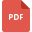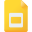# fraction tutorialsDecimals and Fraction Equivalent for Tenths
Decimal and Fractions, Grade 4, United States, MathematicsComparing Decimals and their Arrangement
Mathematics, Grade 4, United States, FractionMultiplication and Division of Fractions
Fraction, Grade 5, United States, MultiplicationAddiing and Subtracting Fractions with Like Denominators
Fraction, Grade 5, United States, MathematicsDividing Fractions by another Fractions
Fraction, Grade 5, United States, MathematicsWorksheet on Fractions with Solutions
Fraction, Grade 4, United States, MathematicsComparing and Ordering Fractions Worksheets
Fraction, Grade 6, United States, MathematicsMultiplying Fractions by Whole Numbers worksheet
Fraction, Grade 6, United States, MathematicsFraction in Highest Terms
Fraction, Grade 5, United States, MathematicsUsing Oder of Operations Procedure with Fractions
Fraction, Grade 6, United States, MathematicsEquivalent Fractions by Multiplication and Division
Fraction, Grade 6, United States, MathematicsFractions Assessment
Basic Math, India, FractionEquivalent Fraction and their Identification
Fraction, Grade 4, United States, MathematicsModel Fraction Word/Story Problems
Mathematics, Grade 3, United States, FractionAdding and Subtracting Fractions Unlike DenominatorsFinding Reciprocals of Fractions and Mixed Numbers
Fraction, Grade 6, United States, MathematicsFraction, Grade 4, United States, MathematicsAddition, Subtraction, Multiplication, Division of Fractions
Fraction, Grade 5, United States, MathematicsMultiplying Fractions Worksheet
Fraction, Grade 8, United States, MultiplicationMultiplying Fractions and Mixed Numbers
Fraction, Grade 5, United States, MultiplicationWorksheet on Order of Operations with Solutions
Fraction, Grade 6, United States, MathematicsBasic Review: Components of a Fraction
Fraction, Grade 4, United States, MathematicsComparing and Ordering Fractions Worksheet
Fraction, Grade 5, United States, MathematicsCompare and Ordering Fractions
Fraction, Grade 3, United States, MathematicsAdding and Subtracting Mixed Numbers and Improper Fractions
Fraction, Grade 3, United States, MathematicsDividing Fractions and Mixed Number
Fraction, Grade 8, United States, DivisionWorksheet on Dividing Fractions
Division, Grade 8, United States, FractionFraction Operations and Examples
Fraction, Grade 5, United States, ArithmeticWorksheet of Fractions and Decimals
Fraction, Grade 3, United States, Decimal and Fractions, MathematicsComparing and Ordering Fractions and Mixed Numbers
Fraction, Grade 6, United States, MathematicsWorksheet on Equivalent fractions
Fraction, Grade 6, United States, MathematicsProper and Improper Fractions
Fraction, Grade 5, United States, MathematicsFractions in Ancient Indian Mathematics
Vedic Mathematics, Class 9, India, FractionReducing Fractions to Lowest Equivalent Form
Fraction, Grade 6, United States, MathematicsAdding and Subtracting Fractions with Sample Questions